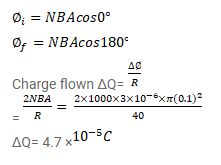# A coil of radius 10 cm and resistance 40Ω has 1000 turns.

Question:

A coil of radius $10 \mathrm{~cm}$ and resistance $40 \Omega$ has 1000 turns. It is placed with its plane vertical and its axis parallel to the magnetic meridian. The coil connected to a galvanometer and is rotated about the vertical diameter through an angle of $180^{\circ}$. Find the charge flows through the galvanometer if the horizontal component of the earth's magnetic field is $B_{H}=3.0 \times 10^{5} \mathrm{~T}$.

Solution: